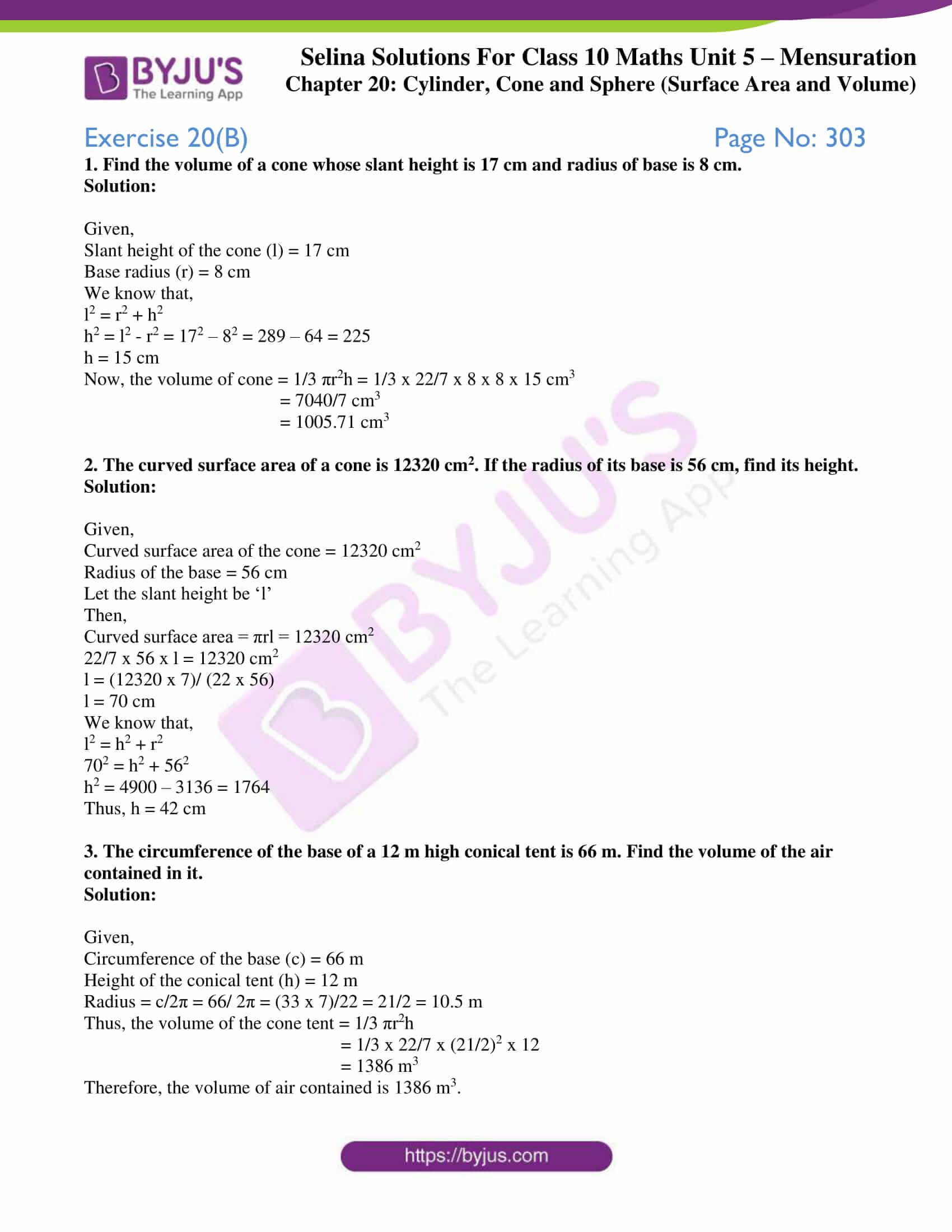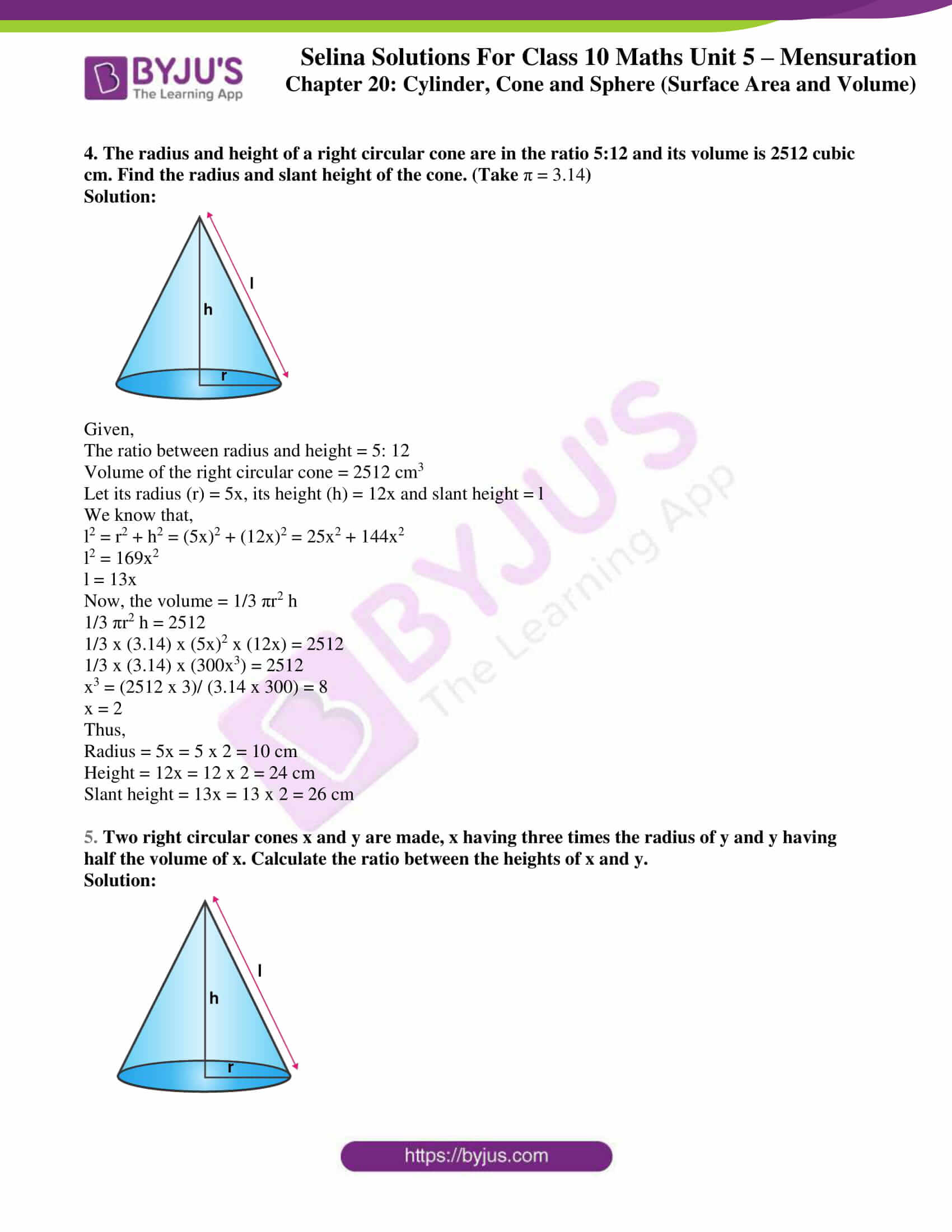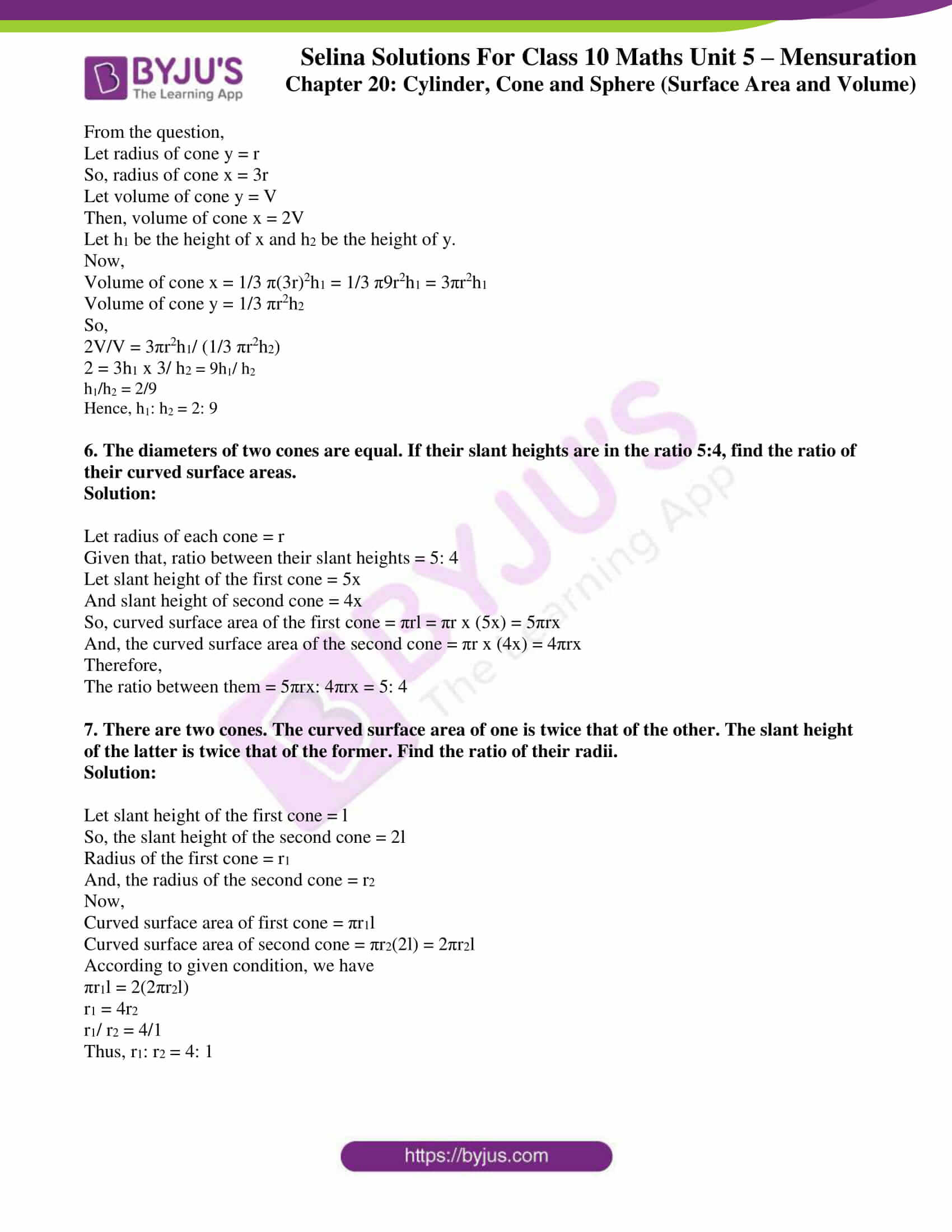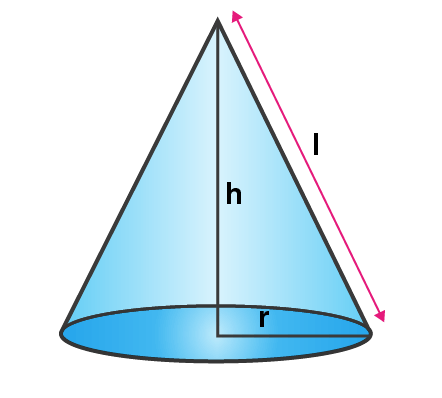# Selina Solutions Concise Maths Class 10 Chapter 20 Cylinder, Cone and Sphere (Surface Area and Volume) Exercise 20B

The solid obtained on revolving a right-angled triangle about one of its sides is called a cone. Problems on finding the surface area (total and curved) and volume of cones are included in this exercise. The Selina Solutions for Class 10 Maths is a very useful resource for students who want to clarify their doubts and learn the correct steps in solving problems, at any time of the day. The Concise Selina Solutions for Class 10 Maths Chapter 20 Cylinder, Cone and Sphere (Surface Area and Volume) Exercise 20(B) PDF is available in the link enclosed below.

## Selina Solutions Concise Maths Class 10 Chapter 20 Cylinder, Cone and Sphere (Surface Area and Volume) Exercise 20(B) Download PDF### Access other exercises of Selina Solutions Concise Maths Class 10 Chapter 20 Cylinder, Cone and Sphere (Surface Area and Volume)

Exercise 20(A) Solutions

Exercise 20(C) Solutions

Exercise 20(D) Solutions

Exercise 20(E) Solutions

Exercise 20(F) Solutions

Exercise 20(G) Solutions

### Access Selina Solutions Concise Maths Class 10 Chapter 20 Cylinder, Cone and Sphere (Surface Area and Volume) Exercise 20(B)

1. Find the volume of a cone whose slant height is 17 cm and radius of base is 8 cm.

Solution:

Given,

Slant height of the cone (l) = 17 cm

Base radius (r) = 8 cm

We know that,

l2 = r2 + h2

h2 = l2 – r2 = 172 – 82 = 289 – 64 = 225

h = 15 cm

Now, the volume of cone = 1/3 πr2h = 1/3 x 22/7 x 8 x 8 x 15 cm3

= 7040/7 cm3

= 1005.71 cm3

2. The curved surface area of a cone is 12320 cm2. If the radius of its base is 56 cm, find its height.

Solution:

Given,

Curved surface area of the cone = 12320 cm2

Radius of the base = 56 cm

Let the slant height be ‘l’

Then,

Curved surface area = πrl = 12320 cm2

22/7 x 56 x l = 12320 cm2

l = (12320 x 7)/ (22 x 56)

l = 70 cm

We know that,

l2 = h2 + r2

702 = h2 + 562

h2 = 4900 – 3136 = 1764

Thus, h = 42 cm

3. The circumference of the base of a 12 m high conical tent is 66 m. Find the volume of the air contained in it.

Solution:

Given,

Circumference of the base (c) = 66 m

Height of the conical tent (h) = 12 m

Radius = c/2π = 66/ 2π = (33 x 7)/22 = 21/2 = 10.5 m

Thus, the volume of the cone tent = 1/3 πr2h

= 1/3 x 22/7 x (21/2)2 x 12

= 1386 m3

Therefore, the volume of air contained is 1386 m3.

4. The radius and height of a right circular cone are in the ratio 5:12 and its volume is 2512 cubic cm. Find the radius and slant height of the cone. (Take π = 3.14)

Solution:Given,

The ratio between radius and height = 5: 12

Volume of the right circular cone = 2512 cm3

Let its radius (r) = 5x, its height (h) = 12x and slant height = l

We know that,

l2 = r2 + h2 = (5x)2 + (12x)2 = 25x2 + 144x2

l2 = 169x2

l = 13x

Now, the volume = 1/3 πr2 h

1/3 πr2 h = 2512

1/3 x (3.14) x (5x)2 x (12x) = 2512

1/3 x (3.14) x (300x3) = 2512

x3 = (2512 x 3)/ (3.14 x 300) = 8

x = 2

Thus,

Radius = 5x = 5 x 2 = 10 cm

Height = 12x = 12 x 2 = 24 cm

Slant height = 13x = 13 x 2 = 26 cm

5. Two right circular cones x and y are made, x having three times the radius of y and y having half the volume of x. Calculate the ratio between the heights of x and y.

Solution:From the question,

Let radius of cone y = r

So, radius of cone x = 3r

Let volume of cone y = V

Then, volume of cone x = 2V

Let h1 be the height of x and h2 be the height of y.

Now,

Volume of cone x = 1/3 π(3r)2h1 = 1/3 π9r2h1 = 3πr2h1

Volume of cone y = 1/3 πr2h2

So,

2V/V = 3πr2h1/ (1/3 πr2h2)

2 = 3h1 x 3/ h2 = 9h1/ h2

h1/h2 = 2/9

Hence, h1: h2 = 2: 9

6. The diameters of two cones are equal. If their slant heights are in the ratio 5:4, find the ratio of their curved surface areas.

Solution:

Let radius of each cone = r

Given that, ratio between their slant heights = 5: 4

Let slant height of the first cone = 5x

And slant height of second cone = 4x

So, curved surface area of the first cone = πrl = πr x (5x) = 5πrx

And, the curved surface area of the second cone = πr x (4x) = 4πrx

Therefore,

The ratio between them = 5πrx: 4πrx = 5: 4

7. There are two cones. The curved surface area of one is twice that of the other. The slant height of the latter is twice that of the former. Find the ratio of their radii.

Solution:

Let slant height of the first cone = l

So, the slant height of the second cone = 2l

Radius of the first cone = r1

And, the radius of the second cone = r2

Now,

Curved surface area of first cone = πr1l

Curved surface area of second cone = πr2(2l) = 2πr2l

According to given condition, we have

πr1l = 2(2πr2l)

r1 = 4r2

r1/ r2 = 4/1

Thus, r1: r2 = 4: 1